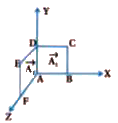Doubtnut App - Get 50 Lakh+ video solutions better than GoogleInstall Now

# A loop, made of straight edges has six corners at A(0,0,0), B(L,0,0) ,C(L,L,0), D(0,L,0), E(0,L,L) and F(0,0,L). A magnetic field →B=B0(ˆi+ˆk)T is present in the region. The flux passing through the loop ABCDEFA (in that order) isSolution in Gujrati

A

B0L2Wb

B

2B0L2Wb

C

2B0L2Wb

D

4B0L2Wb

Text Solution
Verified by Experts

## Consider a plane is made by two loop. Area of loop ABCDA in xy-plane , →A1=|A|ˆk=L2ˆk Area of loop ADEFA in yz- plane →A2=|A|ˆi=L2ˆi Total area of loops are →A=→A1+→A2 ∴→A=L2ˆk+L2ˆi Now flux linked with these loops is ϕ=→A.→B=(L2ˆi+L2ˆk).B0(ˆi+ˆk) where →B=B0(ˆi+ˆk) Magnetic flux , ϕ=→B.→A =B0(ˆi+ˆk).L2(ˆi+ˆk) =B0L2(1+1) =2B0L2